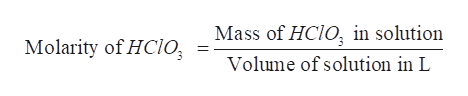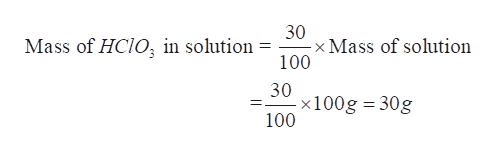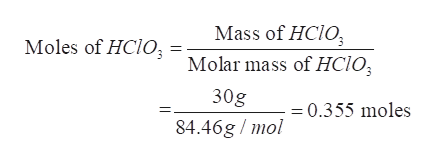The density of a solution that is 30.0 % H C l O3 is 1.167 g/mL. Calculate the molarity of the H C l O3 (molar mass is 84.46 g) solution.HINT : % H C l O3 = [mass H C l O3/mass solution] times 100% and Density = 1.167 g solution / 1 mL solution

Question

The density of a solution that is 30.0 % H C l O3 is 1.167 g/mL. Calculate the molarity of the H C l O3 (molar mass is 84.46 g) solution.
HINT : % H C l O3 = [mass H C l O3/mass solution] times 100% and Density = 1.167 g solution / 1 mL solution

Step 1

Given –

The given solution is 30% HClO3

Density of this solution = 1.167 g/mL

Molar mass of HClO3 = 84.46 g

Thus, the molarity of the HClO3 solution is to be determined.help_outlineImage TranscriptioncloseMass of HCIO, in solution Molarity of HCIO Volume of solution in L fullscreen
Step 2

Step 2-

Let the mass of the solution be 100 g.

The given solution is 30% HClO3 by mass.

Therefore, mass of HClO3 in solution is calculated as-help_outlineImage Transcriptionclose30 x Mass of solution 100 Mass of HCIO in solution = 30 x100g 30g 100 fullscreen
Step 3

Step 3-

Now, mass of HClO3 in solution = 30 g

Molar mass of HClO3 =84.46 g/mol

Therefore, moles of HClO3 can be calculated as number of ...help_outlineImage TranscriptioncloseMass of HCIO Moles of HCIO Molar mass of HC1O 30g =0.355 moles 84.46g /mol fullscreen

Want to see the full answer?

See Solution

Want to see this answer and more?

Our solutions are written by experts, many with advanced degrees, and available 24/7

See Solution
Tagged in

General Chemistry